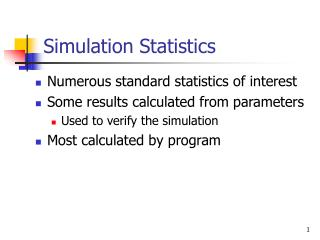# Simulation Statistics - PowerPoint PPT PresentationDownload PresentationSimulation Statistics

Download Presentation## Simulation Statistics

- - - - - - - - - - - - - - - - - - - - - - - - - - - E N D - - - - - - - - - - - - - - - - - - - - - - - - - - -
##### Presentation Transcript

1. Simulation Statistics • Numerous standard statistics of interest • Some results calculated from parameters • Used to verify the simulation • Most calculated by program

2. Some Statistics Average Wait time for a customer = total time customers wait in queue total number of customers Average wait time of those who wait = total time of customers who wait in queue number of customers who wait

3. More Statistics Proportion of server busy time = number of time units server busy total time units of simulation Average service Time = total service time number of customers serviced

4. More Statistics Average time customer spends in system = total time customers spend in system total number of customers Probability a customer has to wait in queue = number of customers who wait total number of customers

5. Traffic Intensity • A measure of the ability of the server to keep up with the number of the arrivals • TI= (service mean)/(inter-arrival mean) • If TI > 1 then system is unstable & queue grows without bound

6. Server Utilization • % of time the server is busy serving customers • If there is 1 server • SU = TI = (service mean)/(inter-arrival mean) • If there are N servers • SU = 1/N * (service mean)/(inter-arrival mean)

7. Statistical Models • Probability: a quantitive measure of the chance or likelihood of an event occurring. • Random: unable to be predicted exactly • In an experiment where events randomly occur but in which we have assigned to each possible outcome a probability, we have determined a probability or stochastic model

8. Terms • Event Space • Event • Complement of an Event • Intersection • Union • Mutually Exclusive

9. Examples • Event Space: The set of all possible events that can occur • ex: {1,2,3,4,5,6} • Event (E): Any single occurrence • ex: E = {4,5} • Complement of E: • Set of all events except E • Ex: Complement of E = {1,2,3,6}

10. Examples • Union: Combination of any 2 event sets • A= {1,2,3} • B = {3,4} • A U B = {1,2,3,4}

11. Examples • Intersection: Overlap of common occurrence of 2 event sets • A= {1,2,3} B = {3,4} • A Π B = {3} • Mutually Exclusive: 2 event sets that have no events in common • A= {1,2} B = {3,4} • A Π B = { }

12. Random variable Practical Definition • a quantity whose value is determined by the outcome of a random experiment

13. Random Variable Examples • X = the number of 4's that occur in 10 rolls • Y = the number of customers that arrive in 1 hour • Z = the number of services that are completed in 5 minutes

14. Discrete vs. Continuous RV EXAMPLE • Discrete: X = number of customers that arrive in 1 hour • Continuous: Y = gallons that flow into the pool in 1 hour • ????: Z = the average age of the customers that arrive in an hour

15. Discrete: Probability Function Let X be a discrete R.V. with possible values x1, x2,…xn. Let P be the probability function P(xi) = (X = xi) such that (a) P(xi) >= 0 for i = 1,2,…n (b) Σ P(xi) = 1

16. Probability FunctionExample • Consider the rolling of a fair die 1/6 for x = 1 P(x) = 1/6 for x = 2 1/6 for x = 3 1/6 for x = 4 1/6 for x = 5 1/6 for x = 6 0 for all other x

17. Cumulative Distribution Function • CDF of a random variable X is F such that F(x) = P (X <= x) • F(X) is continuous • Discrete: sum of probabilities • Continuous: area under the curve

18. Cumulative Distribution Function - Example • Consider the rolling of a fair die 0 for x < 1 1/6 for x < 2 F(X) = 2/6 for x <3 3/6 for x < 4 4/6 for x < 5 5/6 for x < 6 1 for x >= 6

19. Cumulative Function 1 1/2 1/6 • 2 3 4 5 6

20. Discrete vs. Continuous R.V. • Cumulative Distribution Function (CDF) • The CDF of a discrete R.V. X is F such that F(x)= P (X<= x) • Continuous: The CDF of a continuous RV has the properties: • F(x) is continuous, at least piecewise • F(x) exists except in at most a finite number of points

21. Discrete vs. Continuous Random Variables • Random variable: a function whose domain is the event space & whose range is some subset of real numbers • If a random variable assumes a discrete (finite or countably infinite) number of values, it is called a discrete random variable. Otherwise, it is called a continuous random variable.